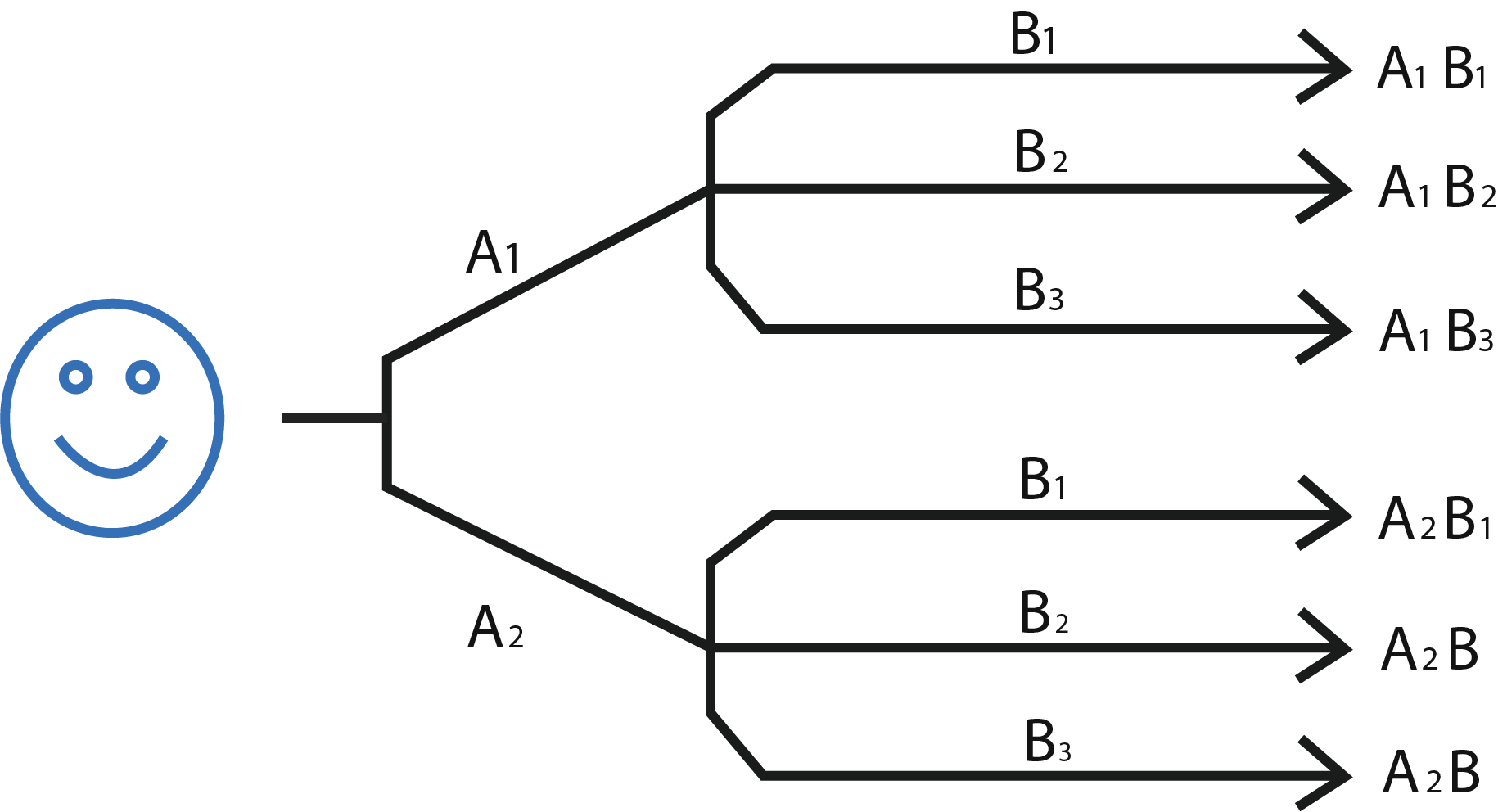Uh Oh! It seems you’re using an Ad blocker!

We always struggled to serve you with the best online calculations, thus, there's a humble request to either disable the AD blocker or go with premium plans to use the AD-Free version for calculators.

Or# Fundamental Counting Principle Calculator

Add the number of choices in the tool and the calculator will calculate the combination

Number of choices for the 1st thing

Number of choices for the 2st thing

Table of Content

Get the Widget!

Add this calculator to your site and lets users to perform easy calculations.

Feedback

How easy was it to use our calculator? Did you face any problem, tell us!

Develop all possible combinations for a specific problem with the help of the fundamental counting principle calculator. This is a useful way to avoid uncertainty in the event of an emergency.

## Definition Fundamental Counting Principle:

“The fundamental counting principle permutations and combinations are used to find the possible alternative of a problem”.

The FPC stands for  Fundamental Counting Principle, you can generate the alternative by the fundamental counting calculator to find a solution.### What is the Fundamental Counting Principle?

The fundamental counting principle is a process of generating the number of alternatives in a situation. In operation management, it is necessary to generate a number of alternatives to perform a task. There may be various situations or a problem that can be handled by the counting principles application.

### Fundamental Counting Principle Formula:

Consider there are “n” ways of doing a certain job and “m” ways of doing the same job.  The fundamental counting principle formula is:

### Fundamental Counting Principle Example:

A restaurant has 5 appetizers, 8 beverages, 9 sweets, and 6 desserts on the menu. Then use the counting principle to find the probability of various combinations of dishes.

#### Solution:

The appetizers and a beverages permutation = appetizers *beverages

The appetizers and a beverages permutation =5*8=40

The beverage and a dessert permutation = beverage*dessert

The beverage and a dessert permutation=8*6=48

The beverage and a dessert and sweet permutation=beverage* dessert *sweet

The beverage and a dessert and sweet permutation=8*6*9= 432

The total combination are =  5 *8 * 9* 6

The total combination =  2160

There can be other permutations and combinations of menus that can be generated by the fundamental counting calculator. The manager can use a combination of dishes to serve clients of various tastes.

## Working of the Fundamental Counting Principle Calculator:

The combination is simple to find by fundamental counting calculator in simple steps:

Let’s see how!

Input:

• Enter the number of choices of first, second, third option, and so on.
• Tap Calculate

Output:

• Total number of permutations

## FAQs:

### Why Use the Counting Principle to Find the Probability?

The fundamental counting principle permutations and combinations are used to generate the alternatives to perform a job in different ways. The multiplication principle for counting provides an alternative to solving a problem.

### What are Two Concepts of Counting Principles?

There are two concepts of the counting principle